×#### Thank you for registering.

One of our academic counsellors will contact you within 1 working day.

Click to Chat

1800-1023-196

+91-120-4616500

CART 0

• 0

MY CART (5)

Use Coupon: CART20 and get 20% off on all online Study Material

ITEM
DETAILS
MRP
DISCOUNT
FINAL PRICE
Total Price: Rs.

There are no items in this cart.
Continue Shopping• Complete JEE Main/Advanced Course and Test Series
• OFFERED PRICE: Rs. 15,900
• View Details

Revision Notes on Introduction to Trigonometry

Trigonometry

To find the distances and heights we can use the mathematical techniques, which come under the Trigonometry. It shows the relationship between the sides and the angles of the triangle. Generally, it is used in the case of a right angle triangle.

Trigonometric RatiosIn a right angle triangle, the ratio of its side and the acute angles is the trigonometric ratios of the angles.

In this right angle triangle ∠B = 90°. If we take ∠A as acute angle then -

AB is the base, as the side adjacent to the acute angle.

BC is the perpendicular, as the side opposite to the acute angle.

Ac is the hypotenuse, as the side opposite to the right angle.

Trigonometric ratios with respect to ∠A

Ratio
Formula
Short form
Value

sin A

P/H
BC/AC

cos A

B/H
AB/AC

tan A

P/B
BC/AB

cosec A

H/P
AC/BC

sec A

H/B
AC/AB

cot A

B/P
AB/BC

Remark

If we take ∠C as acute angle then BC will be base and AB will be perpendicular. Hypotenuse remains the same i.e. AC.So the ratios will be according to that only.
If the angle is same then the value of the trigonometric ratios of the angles remain the same whether the length of the side increases or decreases.
In a right angle triangle, the hypotenuse is the longest side so sin A or cos A will always be less than or equal to 1 and the value of sec A or cosec A will always be greater than or equal to 1.

Reciprocal Relation between Trigonometric Ratios

Cosec A, sec A, and cot A are the reciprocals of sin A, cos A, and tan A respectively.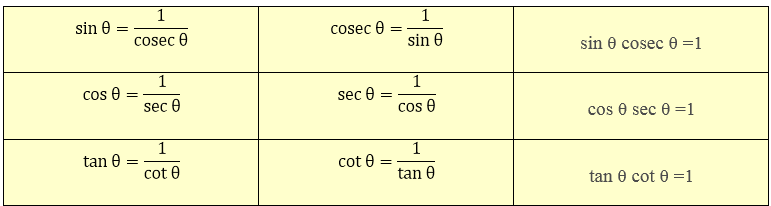uotient RelationTrigonometric Ratios of Some Specific Angles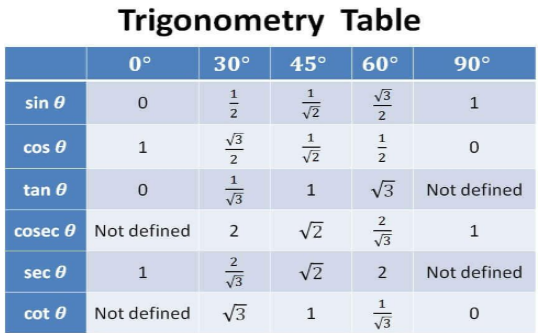Use of Trigonometric Ratios and Table in Solving Problems

Example

Find the lengths of the sides BC and AC in ∆ ABC, right-angled at B where AB = 25 cm and ∠ACB = 30°, using trigonometric ratios.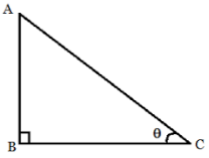Solution

To find the length of the side BC, we need to choose the ratio having BC and the given side AB. As we can see that BC is the side adjacent to angle C and AB is the side opposite to angle C, thereforetan 30°BC = 25√3 cm

To find the length of the side AC, we considerAC = 50 cm

Trigonometric Ratios of Complementary Angles

If the sum of two angles is 90° then, it is called Complementary Angles. In a right-angled triangle, one angle is 90 °, so the sum of the other two angles is also 90° or they are complementary angles.so the trigonometric ratios of the complementary angles will be -

sin (90° – A) = cos A,

cos (90° – A) = sin A,

tan (90° – A) = cot A,

cot (90° – A) = tan A,

sec (90° – A) = cosec A,

cosec (90° – A) = sec A

Trigonometric Identities (Pythagoras Identity)

An equation is said to be a trigonometric identity if it contains trigonometric ratios of an angle and satisfies it for all values of the given trigonometric ratios.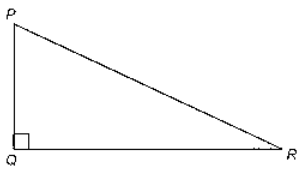In ∆PQR, right angled at Q, we can say that

PQ2 + QR2 = PR2

Divide each term by PR2, we get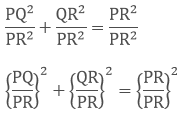(sin R)2 + (cos R)2 = 1

sin2 R + cos2 R =1

Likewise other trigonometric identities can also be proved. So the identities are-

sin2 R + cos2 R = 1

1 + tan2 R = sec2 R

cot2 R + 1 = cosec2 R

How to solve the problems related to trigonometric ratios and identities?

Prove that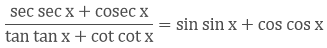L.H.S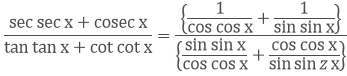(by reciprocal identity and quotient identity)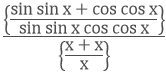(by simplifying the denominator and by Pythagoras identity)Hence, L.H.S = R.H.S### Course Features

• 728 Video Lectures
• Revision Notes
• Previous Year Papers
• Mind Map
• Study Planner
• NCERT Solutions
• Discussion Forum
• Test paper with Video Solution